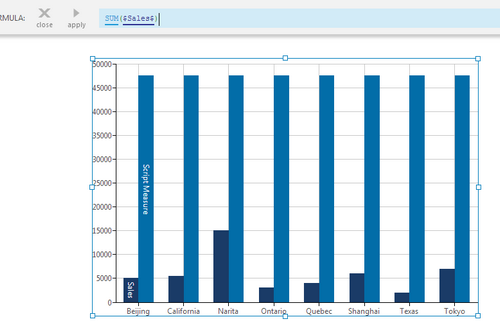# Totals (Sum)

Contents[Hide]

The Sum function calculates the sum total for a set of input values.

## 1. Syntax

```SUM(d0,Alignments...)
```

## 2. Input

The Sum function requires the following input:

• d0 - The set of data values for which the Sum is calculated.

## 3. Parameters

The Sum function accepts the following parameters:

• Alignments... (One or More, Optional) – Hierarchy placeholders to be used as the alignment axis. Input values are grouped by the specified hierarchy values, and the sum calculated for each group: e.g., SUM(\$Quantity\$, \$Country\$) calculates the total for each country.

## 4. Output

The Sum function generates the following output:

• Total - A single value representing the sum total of the input values (or for each group when using alignment parameters).Result displayed as a 2nd series (lighter blue)

Tip
To display the output in a separate visualization such as a data label, you can use a formula visualization.